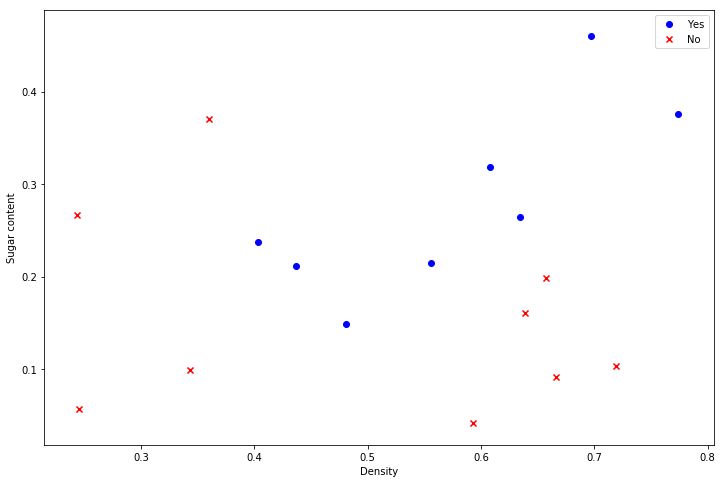《机器学习》 西瓜书的代码实现. 《机器学习》 西瓜书实例 第 6 章

# 《机器学习》西瓜书 第 6 章 编程实例¶

In :
import numpy as np
import pandas as pd
import matplotlib.pyplot as plt

In :
data = pd.read_csv("work/西瓜数据集3.0α.txt")
data

Out:
Density Sugar content Good melon
0 0.697 0.460
1 0.774 0.376
2 0.634 0.264
3 0.608 0.318
4 0.556 0.215
5 0.403 0.237
6 0.481 0.149
7 0.437 0.211
8 0.666 0.091
9 0.243 0.267
10 0.245 0.057
11 0.343 0.099
12 0.639 0.161
13 0.657 0.198
14 0.360 0.370
15 0.593 0.042
16 0.719 0.103
In :
yes = data[data['Good melon'].isin(['是'])]
no = data[data['Good melon'].isin(['否'])]
fig, ax = plt.subplots(figsize=(12, 8))
ax.scatter(yes['Density'], yes['Sugar content'], marker='o', c='b', label='Yes')
ax.scatter(no['Density'], no['Sugar content'], marker='x', c='r', label='No')
ax.legend()
ax.set_xlabel('Density')
ax.set_ylabel('Sugar content')
plt.show()## 线性核与高斯核¶

In :
from sklearn import svm
linear_svc = svm.SVC(kernel='linear')
rbf_svc = svm.SVC(kernel='rbf')

In :
temp = {'是': 1, '否': -1}
X = np.array(data.iloc[:, :2])
y = np.array(data.iloc[:, 2].replace(temp))[None].T

In :
linear_svc.fit(X, y)
rbf_svc.fit(X, y)

/opt/conda/envs/python35-paddle120-env/lib/python3.7/site-packages/sklearn/utils/validation.py:752: DataConversionWarning: A column-vector y was passed when a 1d array was expected. Please change the shape of y to (n_samples, ), for example using ravel().
y = column_or_1d(y, warn=True)
/opt/conda/envs/python35-paddle120-env/lib/python3.7/site-packages/sklearn/utils/validation.py:752: DataConversionWarning: A column-vector y was passed when a 1d array was expected. Please change the shape of y to (n_samples, ), for example using ravel().
y = column_or_1d(y, warn=True)
/opt/conda/envs/python35-paddle120-env/lib/python3.7/site-packages/sklearn/svm/base.py:196: FutureWarning: The default value of gamma will change from 'auto' to 'scale' in version 0.22 to account better for unscaled features. Set gamma explicitly to 'auto' or 'scale' to avoid this warning.
"avoid this warning.", FutureWarning)

Out:
SVC(C=1.0, cache_size=200, class_weight=None, coef0=0.0,
decision_function_shape='ovr', degree=3, gamma='auto_deprecated',
kernel='rbf', max_iter=-1, probability=False, random_state=None,
shrinking=True, tol=0.001, verbose=False)

### 查看支持向量¶

In :
linear_svc.support_vectors_

Out:
array([[0.666, 0.091],
[0.243, 0.267],
[0.343, 0.099],
[0.639, 0.161],
[0.657, 0.198],
[0.36 , 0.37 ],
[0.593, 0.042],
[0.719, 0.103],
[0.697, 0.46 ],
[0.774, 0.376],
[0.634, 0.264],
[0.608, 0.318],
[0.556, 0.215],
[0.403, 0.237],
[0.481, 0.149],
[0.437, 0.211]])
In :
rbf_svc.support_vectors_

Out:
array([[0.666, 0.091],
[0.243, 0.267],
[0.343, 0.099],
[0.639, 0.161],
[0.657, 0.198],
[0.36 , 0.37 ],
[0.593, 0.042],
[0.719, 0.103],
[0.697, 0.46 ],
[0.774, 0.376],
[0.634, 0.264],
[0.608, 0.318],
[0.556, 0.215],
[0.403, 0.237],
[0.481, 0.149],
[0.437, 0.211]])

## SVM 在 iris 数据集上的表现¶

In :
from sklearn import datasets

In :
X = iris['data']
y = iris['target'][None].T

In :
linear_svc.fit(X, y)
rbf_svc.fit(X, y)

/opt/conda/envs/python35-paddle120-env/lib/python3.7/site-packages/sklearn/utils/validation.py:752: DataConversionWarning: A column-vector y was passed when a 1d array was expected. Please change the shape of y to (n_samples, ), for example using ravel().
y = column_or_1d(y, warn=True)
/opt/conda/envs/python35-paddle120-env/lib/python3.7/site-packages/sklearn/utils/validation.py:752: DataConversionWarning: A column-vector y was passed when a 1d array was expected. Please change the shape of y to (n_samples, ), for example using ravel().
y = column_or_1d(y, warn=True)
/opt/conda/envs/python35-paddle120-env/lib/python3.7/site-packages/sklearn/svm/base.py:196: FutureWarning: The default value of gamma will change from 'auto' to 'scale' in version 0.22 to account better for unscaled features. Set gamma explicitly to 'auto' or 'scale' to avoid this warning.
"avoid this warning.", FutureWarning)

Out:
SVC(C=1.0, cache_size=200, class_weight=None, coef0=0.0,
decision_function_shape='ovr', degree=3, gamma='auto_deprecated',
kernel='rbf', max_iter=-1, probability=False, random_state=None,
shrinking=True, tol=0.001, verbose=False)
In :
linear_svc.score(X, y), rbf_svc.score(X, y)

Out:
(0.9933333333333333, 0.9866666666666667)

### 与决策树进行比较¶

In :
from sklearn import tree
clf = tree.DecisionTreeClassifier()

In :
clf.fit(X, y)
clf.score(X, y)

Out:
1.0

## SVR 的训练¶

In :
rbf_svr = svm.SVR(kernel='rbf')
rbf_svr.fit(np.array(data.iloc[:, 0])[None].T, np.array(data.iloc[:, 1])[None].T)

/opt/conda/envs/python35-paddle120-env/lib/python3.7/site-packages/sklearn/utils/validation.py:752: DataConversionWarning: A column-vector y was passed when a 1d array was expected. Please change the shape of y to (n_samples, ), for example using ravel().
y = column_or_1d(y, warn=True)
/opt/conda/envs/python35-paddle120-env/lib/python3.7/site-packages/sklearn/svm/base.py:196: FutureWarning: The default value of gamma will change from 'auto' to 'scale' in version 0.22 to account better for unscaled features. Set gamma explicitly to 'auto' or 'scale' to avoid this warning.
"avoid this warning.", FutureWarning)

Out:
SVR(C=1.0, cache_size=200, coef0=0.0, degree=3, epsilon=0.1,
gamma='auto_deprecated', kernel='rbf', max_iter=-1, shrinking=True,
tol=0.001, verbose=False)
In :
rbf_svr.score(np.array(data.iloc[:, 0])[None].T, np.array(data.iloc[:, 1])[None].T)

Out:
0.035749609530696835